Next: Computing Errors Up: Introduction Previous: Introduction

## The Problems Solved

The primal and dual pair of conic optimization problems over a self-dual cone are defined as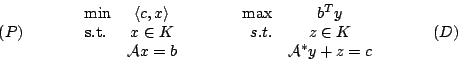where
•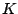is a closed, convex cone in a euclidean space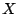.
•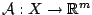is a linear operator, and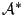is its adjoint.
•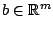, and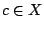.
In the case of a semidefinite-quadratic-linear program these are defined as follows:
• The space: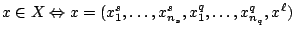, where
•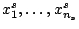are symmetric matrices (possibly of various sizes).
•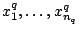are vectors (again, possibly of various sizes).
•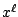is a vector.
• The cone: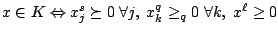, where
•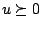means that the symmetric matrix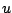is positive semidefinite.
•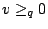means that the vectoris in a quadratic cone (also known as the second-order cone, Lorentz cone or ice cream cone) of appropriate size. That is, if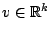, then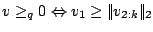.
•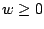means that the vector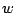is componentwise nonnegative.
• The inner product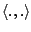: For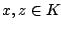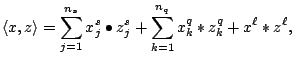where
• For matrices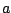and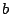,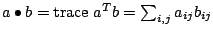.
• For vectorsand,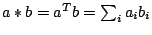.
Thus (P) and (D) become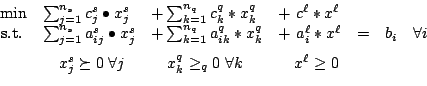(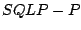)

and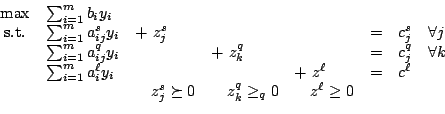(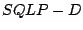)

Thus the feasible set is a product of semidefinite, quadratic and nonnegative orthant cones, intersected with an affine subspace. It is possible that one or more of the three parts of the problem is absent, i.e., any of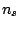,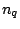, or the length ofmay be zero.

The rotated quadratic cone is defined as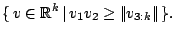It is simply a rotation of the usual quadratic cone, but for the purpose of modeling quadratic inequalities, it is more convenient to use, thus several participating codes support this cone.Next: Computing Errors Up: Introduction Previous: Introduction
Hans D. Mittelmann 2002-08-17